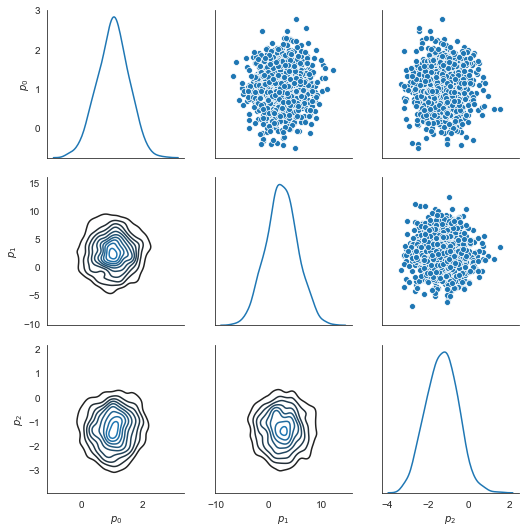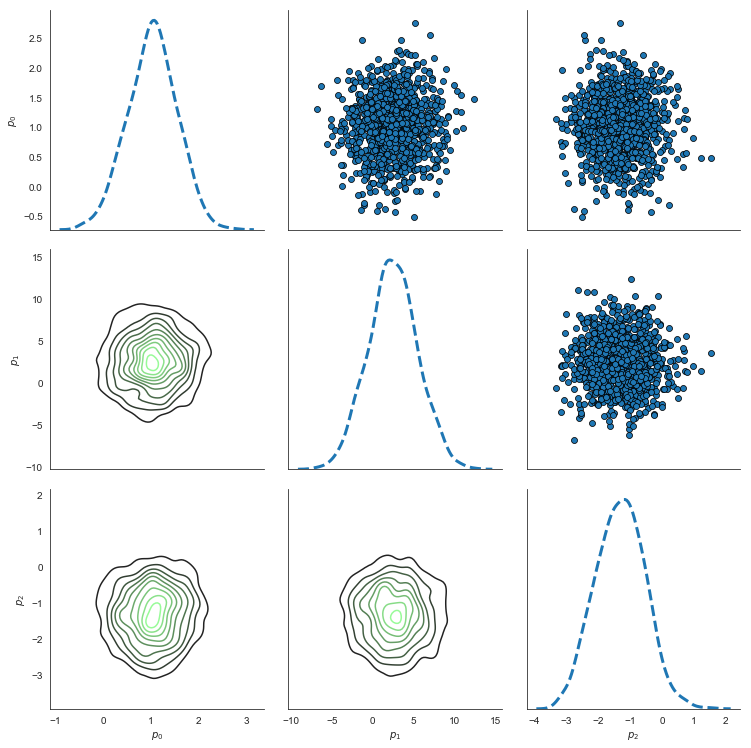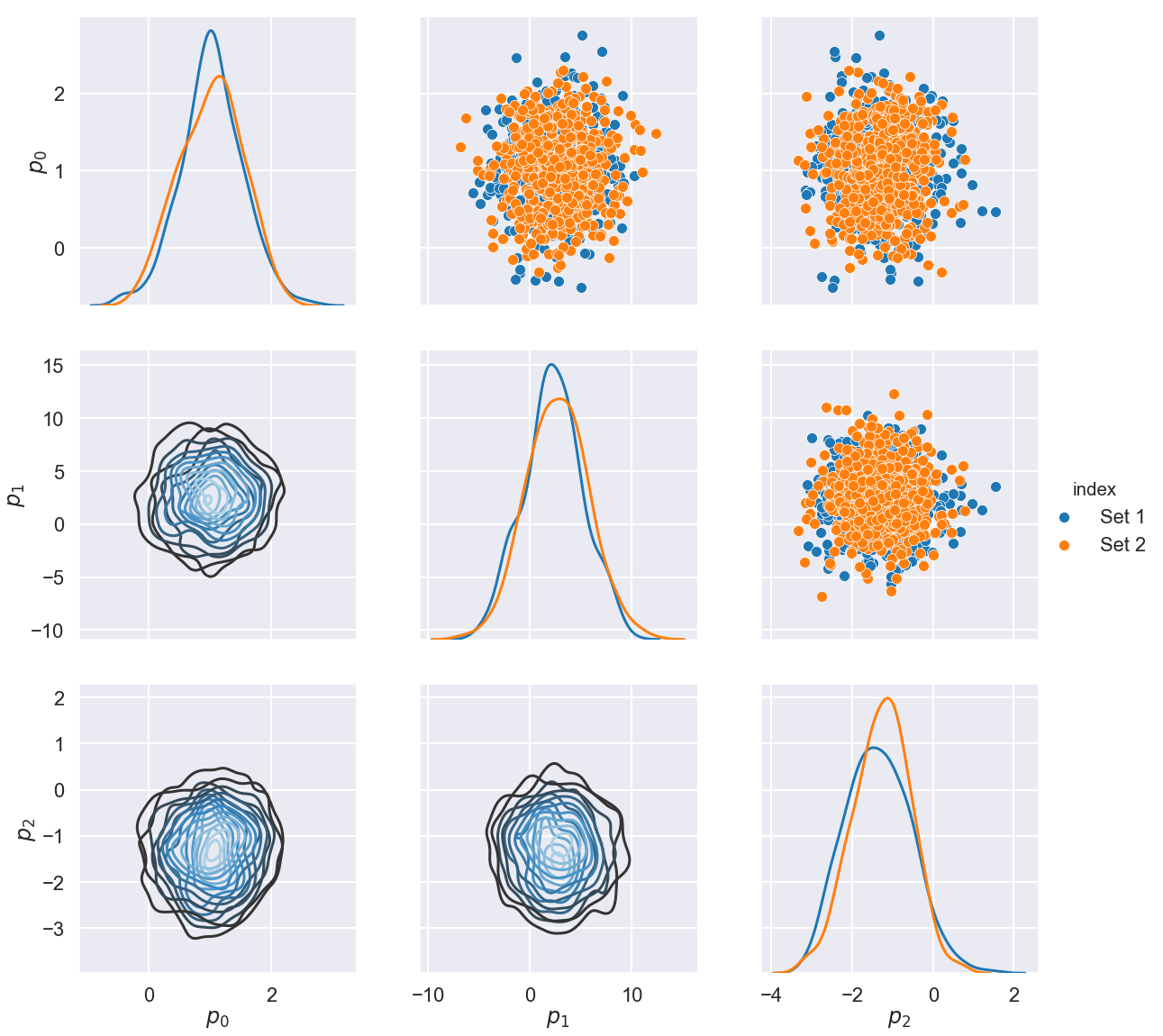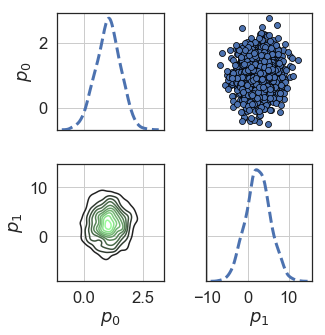# Paired Density Matrix¶

Author(s) Paul Miles | August 7, 2018

This tutorial provides a demonstration of the paired density matrix plotting routine available in mcmcplot.

In :
# import required packages
import numpy as np
from mcmcplot import mcseaborn as mcs
import seaborn as sns
import matplotlib.pyplot as plt
import warnings
warnings.filterwarnings("ignore")


### Generate Random Chains¶

The plotting routines are designed to be used in conjunction with the result of a MCMC simulation. For the purpose of this example, we consider a randomly generated chain. We will consider a chain with 3 parameters that have the following distributions:

• $p_{0} \sim N(1.0, 0.5)$
• $p_{1} \sim N(2.5, 3.0)$
• $p_{2} \sim N(-1.3, 0.75)$
In :
nsimu = 1000
npar = 3
mu = np.array([1.0, 2.5, -1.3])
sig = np.array([0.5, 3.0, 0.75])
chain = np.zeros([nsimu, npar])
for ii in range(npar):
chain[:,ii] = sig[ii]*np.random.randn(nsimu,) + mu[ii]


### Plot Default Paired Density Matrix¶

This routine will generate an array of plots, where the components of the array are as follows:

• Main diagonal: 1D, KDE-plot
• Lower triangle: 2D, KDE-plot
• Upper triangle: scatter-plot (pairwise correlation)

Note, that each function call returns the figure handles as well as the settings used to generate the plots.

In :
fjd, used_settings = mcs.plot_paired_density_matrix(chains = chain, settings = None)
used_settings

Out:
{'skip': 1,
'sns_style': 'white',
'sns': {'figure.facecolor': 'white',
'axes.labelcolor': '.15',
'xtick.direction': 'out',
'ytick.direction': 'out',
'xtick.color': '.15',
'ytick.color': '.15',
'axes.axisbelow': True,
'grid.linestyle': '-',
'text.color': '.15',
'font.family': ['sans-serif'],
'font.sans-serif': ['Arial',
'DejaVu Sans',
'Liberation Sans',
'Bitstream Vera Sans',
'sans-serif'],
'lines.solid_capstyle': 'round',
'patch.edgecolor': 'w',
'patch.force_edgecolor': True,
'image.cmap': 'rocket',
'xtick.top': False,
'ytick.right': False,
'axes.grid': False,
'axes.facecolor': 'white',
'axes.edgecolor': '.15',
'grid.color': '.8',
'axes.spines.left': True,
'axes.spines.bottom': True,
'axes.spines.right': True,
'axes.spines.top': True,
'xtick.bottom': False,
'ytick.left': False},
'pairgrid': {'diag_sharey': False, 'height': 2.5},
'ld_type': <function seaborn.distributions.kdeplot(data, data2=None, shade=False, vertical=False, kernel='gau', bw='scott', gridsize=100, cut=3, clip=None, legend=True, cumulative=False, shade_lowest=True, cbar=False, cbar_ax=None, cbar_kws=None, ax=None, **kwargs)>,
'ld': {},
'md_type': <function seaborn.distributions.kdeplot(data, data2=None, shade=False, vertical=False, kernel='gau', bw='scott', gridsize=100, cut=3, clip=None, legend=True, cumulative=False, shade_lowest=True, cbar=False, cbar_ax=None, cbar_kws=None, ax=None, **kwargs)>,
'md': {},
'ud_type': <function seaborn.relational.scatterplot(x=None, y=None, hue=None, style=None, size=None, data=None, palette=None, hue_order=None, hue_norm=None, sizes=None, size_order=None, size_norm=None, markers=True, style_order=None, x_bins=None, y_bins=None, units=None, estimator=None, ci=95, n_boot=1000, alpha='auto', x_jitter=None, y_jitter=None, legend='brief', ax=None, **kwargs)>,
'ud': {}}### Change the Type of Paired Density Plot, Figure Size, Color, Etc.¶

We can easily adjust the type of paired density plot, size of the figure, and color map. You can specify different types of plots for the main diagonal, lower triangle, and upper triangle elements.

• md_type: Main diagonal plot type.
• ld_type: Lower triangle plot type.
• ud_type: Upper triangle plot type.
In :
pal = sns.dark_palette("palegreen", as_cmap=True)
settings = dict(
pairgrid = dict(height = 3.5),
ld_type = sns.kdeplot,
ld = dict(cmap = pal),
md = dict(lw = 3, linestyle = '--'),
ud = dict(edgecolor = 'black'))
fpg, used_settings = mcs.plot_paired_density_matrix(chains = chain, settings = settings)### Edit the seaborn Style¶

The default seaborn style is white, but it can be changed by defining the sns_style variable. Each style has it's own settings, so see the seaborn style documentation for details. The seaborn package also allows you to set the context. For example, you can set the context for poster which increases the fontsize and contrast for all plot features.

The seaborn package uses pandas data structures, which makes it extremely amenable to categorical data. Below we demonstrate how to add a category to the data structure by sending the index argument to the plot_paired_density_matrix method. For this example, we consider the first half of the chains as "Set 1" and the second half as "Set 2". In an MCMC simulation, you could distinguish the sets based on when the chain appears to have "burned-in".

In :
nsimu = chain.shape
index = []
for ii in range(nsimu):
if ii < nsimu/2:
index.append('Set 1')
else:
index.append('Set 2')
sns.set_context('poster');
settings = {'pairgrid': {'height': 5.5, 'hue': 'index'}, 'ld_type': sns.kdeplot, 'ld': {'cmap': 'Blues_d'}, 'md': {'lw': 3}}
fpg, used_settings = mcs.plot_paired_density_matrix(chains = chain, sns_style='darkgrid',settings = settings, index = index);### Manually Edit Features¶

As seaborn is built on top of matplotlib, you can edit various plot features in the same way. The output from the plot_paired_density_matrix method will contain the figure handle from which all other plot features can be accessed.

In :
pal = sns.dark_palette("palegreen", as_cmap=True)
sns.set_context('paper')
sns.set(font_scale=1.5)
settings = dict(
sns = {'axes.grid': True},
pairgrid = dict(despine = False), # adds right and top borders back to plot
ld = dict(cmap = pal),
md = dict(lw = 3, linestyle = '--'),
ud = dict(edgecolor = 'black'))
fpg, used_settings = mcs.plot_paired_density_matrix(chains = chain[:,0:2], sns_style = 'white', settings = settings)
fpg.savefig('basic_paired_density_matrix.png')